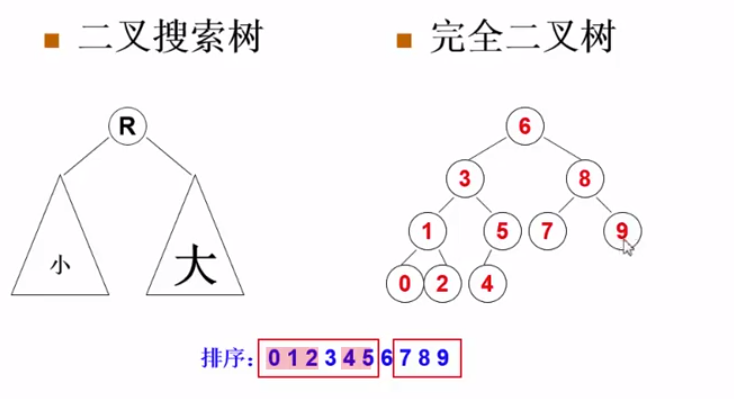Scott's world.

# Complete Binary Search Tree

Word count: 567Reading time: 3 min
2019/08/02 Share# Complete Binary Search Tree

A Binary Search Tree (BST) is recursively defined as a binary tree which has the following properties:

• The left subtree of a node contains only nodes with keys less than the node’s key.

• The right subtree of a node contains only nodes with keys greater than or equal to the node’s key.

• Both the left and right subtrees must also be binary search trees.

A Complete Binary Tree (CBT) is a tree that is completely filled, with the possible exception of the bottom level, which is filled from left to right.

Now given a sequence of distinct non-negative integer keys, a unique BST can be constructed if it is required that the tree must also be a CBT. You are supposed to output the level order traversal sequence of this BST.

### Input Specification:

Each input file contains one test case. For each case, the first line contains a positive integer N (≤1000). Then N distinct non-negative integer keys are given in the next line. All the numbers in a line are separated by a space and are no greater than 2000.

### Output Specification:

For each test case, print in one line the level order traversal sequence of the corresponding complete binary search tree. All the numbers in a line must be separated by a space, and there must be no extra space at the end of the line.

## 题意分析

• 给一串构成树的序列，已知该树是完全二叉搜索树，求它的层序遍历的序列• 因为是二叉搜索树,所以将数组排序即可得到中序序列

• 根据完全二叉树的特点也易得到左子树的规模,得到后即可得到根结点在中序数组中的下标,因此可以通过递归得到左右子树,最后通过T数组输出层序遍历